Home MonkeyNotes Printable Notes Digital Library Study Guides Study Smart Parents Tips College Planning Test Prep Fun Zone Help / FAQ How to Cite New Title Request

5.13 Rate Measure (Distance, Velocity and Acceleration)

If y = s (t) represents the position function, then y = s (t) represents the instantaneous

velocity i.e.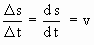and a = v(t) = s" (t) represents the

instantaneous acceleration at time t i.e. a =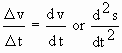Note that a =(1) If v > 0 (i.e. positive velocity) indicates that the position is increasing with increasing time while v < 0 (i.e. negative velocity) indicates the decreasing position as time decreases.

(2) If v = 0 implies that distance s remains constant on the given interval of time.

(3) If a > 0 implies that velocity is increasing with respect to time and a < 0 implies that the velocity is decreasing with respect to time.

(4) If v = constant on an interval of time, then a = 0 on that interval.

Example 36 A particle is moving in a straight line so that after t seconds its distance s from a fixed point O on the line is given by s (t) = 8t + t3.

Find (i) the velocity at time t and also t = 2

(ii) the initial velocity

(iii) acceleration at t and also at t = 2

Solution : s (t) = 8t + t3

(i) v =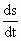= 8 + 3t2

\ velocity at (t = 2) = 8 + 12 = 20 unit /s

(ii) Putting t = 0, initial velocity = 8 + 3 ´ 0 = 8 unit /s

(iii) Acceleration a =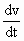= 6t

\ acceleration (at t = 2) = 6 ´ 2 = 12 unit /s2

Example 37 The position of a particle on a line is a given by s (t) = 2t3 - 9t2 + 12t . Calculate its acceleration when it stops.

Solution : s (t) = 2t3 - 9t2 + 12t

\ v == 6t2 - 18t + 12 and a == 12t - 18

When the particle stops, its velocity (v) = 0

\ 6 (t2 - 3t + 2) = 0 Þ (t - 2) (t - 1) = 0

\ t = 1 and 2

When t = 1 a = 12 (1) - 18 = - 6 unit /s2 and

When t = 2 a = 12 (2) - 2 = 6 unit /s2Your browser does not support the IFRAME tag.

Example 38 A point moves in accordance with the law v (t) = a + bt + ct2 and the initial velocity and acceleration are 3 and 2 respectively. Also the acceleration at the end of 1st second is 12. Find the velocity at the end of 3 seconds and the acceleration at the end of 4 seconds.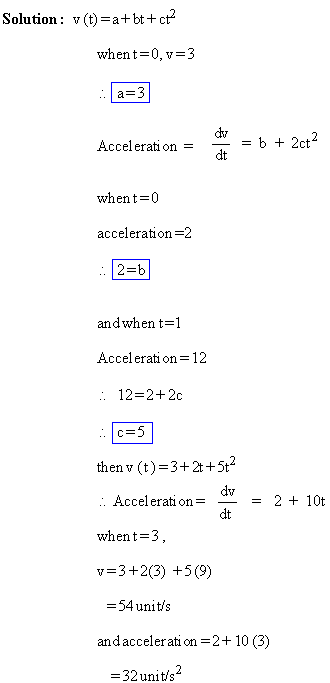Index